# Confirmation and generalization of my solution to the problem of the cosmological constant ??

## Recommended Posts

Hi,

I proposed a mathematical solution to the cosmological constant problem. However, I have not found a physical explanation. Failing that, I found a generalization of this solution to the whole universe to validate a hypothesis that had been made in this solution

In this it is, it seems to me, a confirmation (and perhaps help to understand the problem of the cosmological constant?).

The energy density of the quantum vacuum in Planck units is:

$A=m_pc^2/l_p^3=\hbar(l_p^{-2})^2.c$

I, on the other hand, found this unknown hypothetical quantum energy density of cosmological constant :

$B=\frac{1}{(8\pi)^2}\hbar(\Lambda_{m^{-2})^2.c}$

and demonstrated that the cosmological constant

$C= \sqrt{\hbar(l_p^{-2})^2.c} \sqrt{ \frac{1}{(8\pi)^2} \hbar(\Lambda_{m^{-2 }})^2.c}=\sqrt{A} \sqrt{B}$

Let's consider $H_0$ the Hubble parameter (or Hubble constant) in $s^{-1}$.

We want a dimension in $L^{-2}$ to replace $\Lambda_{m^{-2}}$.

So we'll write $H_0 c^{-2}$ instead of $\Lambda_{m^{-2}}$ to get $B'=\frac{1}{(8\pi)^2} \hbar (H_0/c^2)^2.c$, "an energy density of Planck's universe for $H_0$".

Let's consider

$\rho_c=\frac{3 c^2 H_0^2}{8\pi G}$ , the critical density of the universe for $H_0$.

We have

$3 \sqrt{A} \sqrt{B'}=\rho_c$

The method of dimensional analysis for application in quantum mechanics of general relativity data operates again...

Edited by stephaneww
##### Share on other sites

oops error  in the first post :

Read $H_0^2 c^{-2}$ instead of $H_0 c^{-2}$

Let's consider $H_0$ the Hubble parameter (or Hubble constant) in $s^{-1}$.

We want a dimension in $L^{-2}$ to replace $\Lambda_{m^{-2}}$.

So we'll write $H_0^2 c^{-2}$ instead of $\Lambda_{m^{-2}}$ to get $B'=\frac{1}{(8\pi)^2} \hbar (H_0^2/c^2)^2.c$, "an energy density of Planck's universe for $H_0$".

Let's consider $\rho_c=\frac{3 c^2 H_0^2}{8\pi G}$ the critical density of the universe for $H_0$.

We have $3 \sqrt{A} \sqrt{B'}=\rho_c$

for a quick demonstration we'll use $A=\frac{c^7}{G^2 \hbar}=m_p c^2/l_p^3 \text{ } J/m^3$

Edited by stephaneww
##### Share on other sites

I see one problem the critical density has three contributors. Matter radiation and Lambda.

Currently the primary contributor to expansion being Lambda. However this isn't always the case

Critical density is normalized with the other three cosmological parameters a portion of the critical density. Though our universe is perfectly critically dense it's close enough for a good approximation.

You need to derive which portion of critical density is due to the cosmological constant.

Edited by Mordred
##### Share on other sites

Hello, Mordred.

I believe you're asking what we covered on pages one and two of this thread, aren't you?

Otherwise I don't know what you're talking about for the moment, can you please specify (here I parallel the evolution of the total  critical density with the approach of my previous solution : square root of Planck's energy density)

##### Share on other sites

Yes we covered this in those pages. Recall that critical density is a calculated value that does not represent the actual total density.  It also does not separate the three contributors to expansion.

Though our universe is close to the critical density value you still have the contributions of matter, radiation and Lambda. The first two vary in contribution as the universe expands while the cosmological constant does not.

On the first page I posted a specific formula to calculate the energy density as a function of Z for the former two...

Edited by Mordred
##### Share on other sites

7 hours ago, Mordred said:

Yes we covered this in those pages. Recall that critical density is a calculated value that does not represent the actual total density.  It also does not separate the three contributors to expansion.

Okay, thanks.

My question is: can this new application of dimensional analysis of my hypothetical value $B$ in QM of the cosmological constant be validly duplicated for $B'$ with the  Hubble's constant ?

In view of the result, I think yes.

Otherwise for the actual measurement of the critical density, it seems to me that at each measurement, we are either very slightly above or very slightly below.

Edited by stephaneww
##### Share on other sites

Well you know the Hubble constant isn't constant. It's value changes as the universe evolves.

Look at the H/H_0 column on the table in the previous thread on the first page.

Now if H varies in time then applying the critical density formula it too must vary in time.

Yet the cosmological constant is constant in time...so does the curvature term k.

Edited by Mordred
##### Share on other sites

1 hour ago, Mordred said:

Now if H varies in time then applying the critical density formula it too must vary in time.

This is the case

we find the formula of the critical density (*c^2 to have J/m^3) with a factor 3, so it works with the evolution of $H$ in time

The method for finding $B'$ (Hubble constant) is the same as that used to determine the hypothetical $B$ (cosmological constant) value here: https://www.scienceforums.net/topic/122453-an-attempt-to-approach-a-notion-of-solubility-in-cosmology-to-explain-the-cosmological-constant/

In short, the method seems reproducible. My question therefore concerns the validity of this hypothesis.

demonstration :

$A=\frac{c^7}{G^2 \hbar}$

$B'=\frac{1}{(8 \pi)^2}(\frac{H^2}{c^2})^2\hbar c$

$A B'=\frac{c^8}{(8 \pi)^2 G^2}\frac{H^4}{c^4}$

$A B'=\frac{c^4 H^4 }{(8 \pi)^2 G^2}$

$3 \sqrt{A }\sqrt{B'}=\frac{3 H^2 c^2}{8 \pi G} = \rho_c c^2$

(the c^2 factor for the critical density differs between the French and English versions of wikipedia, hence a small float on this point)

Edited by stephaneww
##### Share on other sites

Well you can test it I finally got a new link to the updated cosmocalculator. Looks like they added a lot of flexibility to it

They have the Omega columns now for the portion each contribute of the total density parameter and how each evolved over time.

I would suggest testing your model to it.

${\scriptsize\begin{array}{|r|r|r|r|r|r|r|r|r|r|r|r|r|r|r|r|} \hline z&T (Gy)&R (Gly)&D_{now} (Gly)&Temp(K) \\ \hline 1.09e+3&3.72e-4&6.27e-4&4.53e+1&2.97e+3\\ \hline 3.39e+2&2.49e-3&3.95e-3&4.42e+1&9.27e+2\\ \hline 1.05e+2&1.53e-2&2.34e-2&4.20e+1&2.89e+2\\ \hline 3.20e+1&9.01e-2&1.36e-1&3.81e+1&9.00e+1\\ \hline 9.29e+0&5.22e-1&7.84e-1&3.09e+1&2.81e+1\\ \hline 2.21e+0&2.98e+0&4.37e+0&1.83e+1&8.74e+0\\ \hline 0.00e+0&1.38e+1&1.44e+1&0.00e+0&2.73e+0\\ \hline -6.88e-1&3.30e+1&1.73e+1&1.12e+1&8.49e-1\\ \hline -8.68e-1&4.79e+1&1.74e+1&1.43e+1&3.59e-1\\ \hline -9.44e-1&6.28e+1&1.74e+1&1.56e+1&1.52e-1\\ \hline -9.76e-1&7.77e+1&1.74e+1&1.61e+1&6.44e-2\\ \hline -9.90e-1&9.27e+1&1.74e+1&1.64e+1&2.73e-2\\ \hline \end{array}}$

There is still some bugs in the new calc that has occurred due to having to change the host site. They are still working on it

Edited by Mordred
##### Share on other sites

Hum, I don't known if that calculator is ok for all datas : for example H input (=67.74) isn't H output, and there's a little discrepancy I don't understand about rho.

$${\scriptsize\begin{array}{|r|r|r|r|r|r|r|r|r|r|r|r|r|r|r|r|} \hline z&T (Gy)&H(t)&rho, kg/m^3&OmegaL&OmegaT \\ \hline 1.09e+3&3.70793e-4&1.55837e+6&4.61214e-18&1.28867e-9&1.00e+0\\ \hline 3.39e+2&2.48188e-3&2.47284e+5&1.16132e-19&5.11790e-8&1.00e+0\\ \hline 1.05e+2&1.52326e-2&4.16481e+4&3.29421e-21&1.80424e-6&1.00e+0\\ \hline 3.20e+1&8.97471e-2&7.17048e+3&9.76466e-23&6.08678e-5&1.00e+0\\ \hline 9.29e+0&5.20128e-1&1.24469e+3&2.94228e-24&2.02004e-3&1.00e+0\\ \hline 2.21e+0&2.96640e+0&2.23251e+2&9.46559e-26&6.27909e-2&1.00e+0\\ \hline 0.00e+0&1.37737e+1&6.74009e+1&8.62765e-27&6.88894e-1&1.00e+0\\ \hline -6.88e-1&3.29612e+1&5.63238e+1&6.02483e-27&9.86506e-1&1.00e+0\\ \hline -8.68e-1&4.78813e+1&5.59720e+1&5.94981e-27&9.98945e-1&1.00e+0\\ \hline -9.44e-1&6.28349e+1&5.59450e+1&5.94406e-27&9.99911e-1&1.00e+0\\ \hline -9.76e-1&7.77911e+1&5.59427e+1&5.94357e-27&9.99993e-1&1.00e+0\\ \hline -9.90e-1&9.27477e+1&5.59428e+1&5.94360e-27&9.99988e-1&1.00e+0\\ \hline \end{array}}$$

Edited by stephaneww
##### Share on other sites

The Omega total is default normalized to critical density of 1. The calc allows you to change this in the inputs along with H. This gives flexibility to use it with different datasets.

Also allows you to change the curvature term. I'm still learning the new features so I will be comparing it to other datasets to see how accurate it is overall. (Keep in mind sometimes the number of digits on the inputs will matter on rounding errors.)

##### Share on other sites

I confess I don't really understand. rho depends theoretically only on H? but we don't find it for H input nor for H ouput.

7 minutes ago, Mordred said:

The Omega total is default normalized to critical density of 1

I kept this value

##### Share on other sites

IsThe total energy/mass density doesn't just depend on H. It also involves the mass density for matter, radiation and Lambda. Radiation and matter will stretch differently due to expansion However the mean average wavelength stretch will become  equal at Z_eq. (Matter, radiation equality).

Then too you must also equate the equations of state for matter and radiation to determine the actual energy density.

Unfortunately someone changed this page and gave the Lambda approximation for the universe today however during matter domination or radiation dominant eras you would use the applicable equation of state. As an approximation. It is more precise to use the density of each at the Z being examined. (The scale factor can also be used as it equates and does help simplify the calculations) however option works

The critical density formula is in actuality a matter only approximation. The pressure equals zero.

This isn't true for radiation or Lambda.

Edited by Mordred
##### Share on other sites

1 hour ago, Mordred said:

IsThe total energy/mass density doesn't just depend on H. It also involves the mass density for matter, radiation and Lambda. Radiation and matter will stretch differently due to expansion However the mean average wavelength stretch will become  equal at Z_eq. (Matter, radiation equality).

Okay, if the radiation is missing from rho, it makes the cross-reference a lot harder to check.(if I understand what you are saying).

For H=67.74 (input) I have rho_c=8.61916 10^-27 kg/m^3, against  rho_c=8.62765 10^-27 kg/m^3 in the calculator

1 hour ago, Mordred said:

Then too you must also equate the equations of state for matter and radiation to determine the actual energy density.

I'm really uncomfortable with state equations.🙄

Edited by stephaneww
##### Share on other sites

On 7/11/2020 at 5:55 PM, Mordred said:

The critical density formula is in actuality a matter only approximation. The pressure equals zero.

we can give it the dimension of a pressure by multiplying by c^2

##### Share on other sites

No that doesn't give matter a pressure term.

##### Share on other sites

17 minutes ago, Mordred said:

No that doesn't give matter a pressure term.

how to explain then that rho_c * c^2 = [M L^-1 T^-2] has the dimension of a pressure?

where is the error?

Edited by stephaneww
##### Share on other sites

You will be applying pressure to something that in cosmological observation doesn't exert pressure. This would give you the wrong equation of state for matter.

Just because you can mathematically do something doesn't necessarily mean it's correct...

Edited by Mordred
##### Share on other sites

• 2 months later...

Hello.

The weak point of my mathematical solution is that it does not allow for physical interpretation.

Below I will try to propose an interpretation of the cosmological constant problem :

The cosmological constant problem as a drag coefficient. ?

Having read "the particles of a perfect gas have speeds close to those of light" and knowing that the formula of the cosmological constant can be interpreted as the expression is of a perfect fluid at negative pressure during my research on search engines I found the notion of drag coefficient .

Its definition and its dimension seemed interesting enough to me to make a numerical verification with the data / formulas that I use or propose:

Definition of the drag coefficient :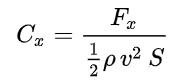adimensioned.

With :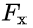= Planck force ( = c4/G )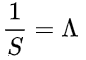because the cosmological constant has the dimension of the inverse of a area.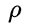density in Planck units. (mp/lp3, Kg/m3)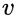take here the value of c, speed of light (speed of "agitation" assumed from the pressure of the cosmological constant),

In the end we get this calculation of the vacuum catastrophe with Planck's values :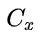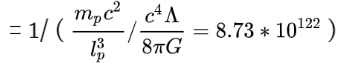I think within a factor of 16 pi.

Note: we can formulate  the cosmological constant problem indifferently with zero-point energy or Planck's units, the order of magnitude is the same.

Is it a possible interpretation ?

Edited by stephaneww

## Create an account

Register a new account